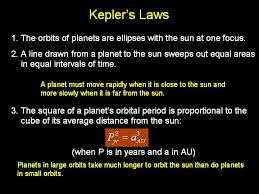## Posts

Showing posts from July, 2014

### What is proof of Kepler law of planetary motion that all planet has elliptical orbit? And why they have elliptical orbit instead of a circular orbit?

The orbit of a planet is an ellipse where one focus of the ellipse is the sun.
An ellipse is defined by two focii and all points for which the sum of the distances are the same. The semimajor axis (a) is the long distance from the center to edge of the ellipse. If r1 and r2 are the distances from the focii to any point on the ellipse then r1 + r2 = 2a. The short axis is called the semiminor axis.

How “elliptical” an orbit is can be described by the eccentricity(e). The eccentricity is equal to the distance between a focus and the center (c) of the ellipses divided by the semimajor axis (a). That is, e = c/a.
See the elementary proof: View

### What multiple of distance between the centre of the earth and that of the moon is the distance between the centre of the earth and the geostationary satelite which always stays above the fixed location on the equator ? take the cycle of revolution of the moomn around the earth to be 27 days ?According to Kepler’s third law of planetary motion T2=R3 ( For geo stationary satellite)-------1 T’2=R’3( For Moon)----------------------------2 Dividing eq(2) by eq(1), we get T’2 / T2=R’3/ R3 Since T’=27 T Then we can write as 27 T2 /T2= R’3/ R3 Or R’3=27 R3 Or
R’=3 R or R= 1/3 R' so the distance between the centre of the earth and the geostationary satellite which always stays above the fixed location on the equator is 1/3 times distance between the centre of the earth and that of the moon .